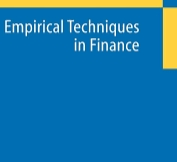## Posts

Showing posts with the label summarize-definition

### RISK ANALYSIS TECHNIQUES FOR TRADERS- The Empirical TechniquesRISK ANALYSIS TECHNIQUES FOR TRADERS The Empirical Techniques TO SUMMARIZE THUS FAR You have seen that a good system is the one with the highest geometric mean. Yet to find the geometric mean you must know f. You may find this confusing. Here now is a summary and clarification of the process: Take the trade listing of a given market system. 1. Find the optimal f, either by testing various f values from 0 to 1 or through iteration. The optimal f is that which yields the highest TWR. 2. Once you have found f, you can take the Nth root of the TWR that corresponds to your f, where N is the total number of trades. This is your geometric mean for this market system. You can now use this geometric mean to make apples-to-apples comparisons with other market systems, as well as use the f to know how many contracts to trade for that particular market system. Once the highest f is found, it can readily be turned into a dollar amount by dividing the biggest loss